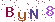javascript的四舍五入函数
2012-3-25 11:59 Sunday

js中只有对整数四舍五入的函数，想要精确到小数点后面的位数，则只能自己写了。

```function roundNum(num,length) {
return Math.round(num*Math.pow(10,length))/Math.pow(10,length);
}  ```

Math.pow(x,y)是计算x的y次方。

Math.ceil求最小的整数但不小于本身。

Math.round求本身的四舍五入。

Math.floor求最大的整数但不大于本身。

```function toFixed( [fractionDigits : Number] ) : String
fractionDigits

toFixed 方法返回一个字符串，该字符串表示一个以定点表示法表示的数字。 该字符串中有效数的小数点之前包含一位数字，而小数点后必须包含 fractionDigits 位数字。

Number 对象```
+1 10Github